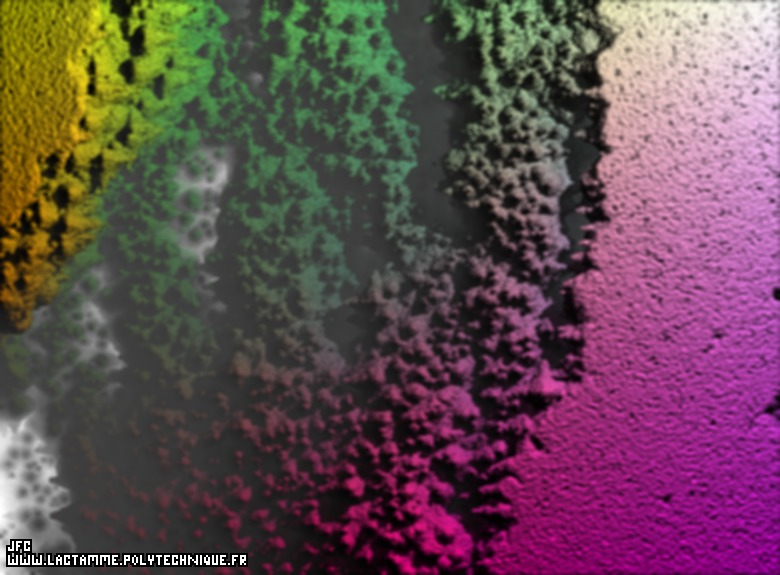Close-up on a pseudo-octonionic Mandelbrot set (a 'Mandelbulb') transformed using the tridimensional life game -tridimensional cross-section- [Agrandissement d'un ensemble de Mandelbrot dans l'ensemble des pseudo-octonions (un 'Mandelbulb') transformé en utilisant le jeu de la vie tridimensionnel -section tridimensionnelle-].

This Mandelbrot set is a tridimensional cross-section and was computed with a polynomial 'P' of the first degree ('C' denoting the current octonionic point) and the following eight functions:
```
P(o) = 1*o + C
```
```
8
fR(R ,R ) = (R *R )
1  2      1  2
```
```
fA1(A1 ,A1 ) = 8*(A1 +A1 )
1   2         1   2
```
```
fA2(A2 ,A2 ) = 8*(A2 +A2 )
1   2         1   2
```
```
fA3(A3 ,A3 ) = 8*(A3 +A3 )
1   2         1   2
```
```
fA4(A4 ,A4 ) = 1*(A4 +A4 )
1   2         1   2
```
```
fA5(A5 ,A5 ) = 1*(A5 +A5 )
1   2         1   2
```
```
fA6(A6 ,A6 ) = 1*(A6 +A6 )
1   2         1   2
```
```
fA7(A7 ,A7 ) = 1*(A7 +A7 )
1   2         1   2
```

See the same object without the transformation:The bidimensional life game was initially defined by Conway. It uses an empty square mesh (all vertices are turned off). At time t=0 some vertices are occupied (they are turned on): this is the initial state. To go from the time t to the time t+1, it suffices to count for each vertex -or "Cell"- C(x,y) the number N of its neighbours (it is less than or equal to 3^2-1=8) and then to possibly change the state of M according to the following bidimensional automata rules:
```                    [R1 = Birth]        ((C(t).IS.off).AND.(N == 3))            ==> C(t+1) on
[R2 = Death]        ((C(t).IS.on).AND.((N < 2).OR.(N > 3))) ==> C(t+1) off
[R3]                other cases ==> C(t+1)=C(t)
```
The boundary conditions can be periodical or not.

This process can extended in a tridimensional space. The number N of neighbours of the vertex -or "Cell"- C(x,y,z) is computed (it is less than or equal to 3^3-1=26) and the preceding rules can be extended as follows:
```                    [R1 = Birth]        ((C(t).IS.off).AND.((N >= NB1).AND.(N <= NB2))) ==> C(t+1) on
[R2 = Death]        ((C(t).IS.on).AND.((N < ND1).OR.(N > ND2)))     ==> C(t+1) off
[R3]                other cases ==> C(t+1)=C(t)
```
The bidimensional and tridimensional processes can be extended one step further using two binary lists 'LD' and 'LA' ("Dead" -off- and "Alive" -on- respectively):
```                    [R1 = Birth]        ((C(t).IS.off).AND.(LD[N] == 1))  ==>C(t+1)=on
[R2 = Death]        ((C(t).IS.on).AND.(LA[N] == 1))   ==>C(t+1)=off
[R3]                other cases ==> C(t+1)=C(t)
```
("1" means "to change the state" and "0" means "the state is unchanged").

For this picture, the parameters have the following values:
```                    LD="000010001110000011100010000"
LA="111111100011111110000111111"
```

See some related pictures (including this one):[Plus d'informations à propos des Ensembles Fractals Déterministes N-Dimensionnels (en français/in french)]

(CMAP28 WWW site: this page was created on 03/13/2012 and last updated on 05/12/2021 19:12:34 -CEST-)

[See the generator of this picture [Voir le générateur de cette image]]

[See all related pictures (including this one) [Voir toutes les images associées (incluant celle-ci)]]

[See the following comment(s): octonionic numbers, pseudo-octonionic numbers, Mandelbrot set [Voir le(s) commentaire(s) suivant(s): octonions, pseudo-octonions, ensemble de Mandelbrot]]

[Go back to AVirtualMachineForExploringSpaceTimeAndBeyond [Retour à AVirtualMachineForExploringSpaceTimeAndBeyond]]

[The Y2K Bug [Le bug de l'an 2000]]

[Site Map, Help and Search [Plan du Site, Aide et Recherche]]
[Mail [Courrier]]
[About Pictures and Animations [A Propos des Images et des Animations]]